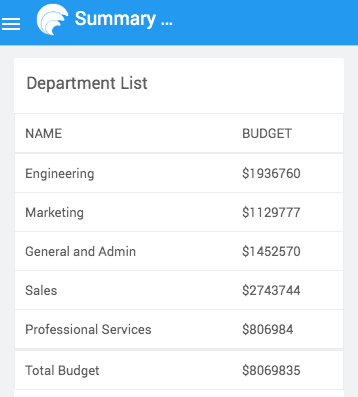Version: v11.4.3

# Data Table - Summary Row Function

When creating a data table, you can use Summary Row Function to add a summary at the bottom of the table. This summary computes on the client-side or the server-side.

Using Summary Row Function, you can summarize any number of columns. A table can also include more than one summary row. To calculate the summary, you can simply use in-built javascript functions like `sum, minimum, maximum, percent`. Application developers may also write their own javascript function that calculates the summary.

You can set summary row using the below-exposed function on a column in the Beforedatarender event callback:

• `setSummaryRowData`

## setSummaryRowData​

WaveMaker provides aggregate functions that can be referenced and used in the `setSummaryRowData` method for using inbuilt aggregate functions for DataTable columns. This method accepts a single or an array of:

• Plain values and Built-in aggregation functions
• Custom Functions with aggregation logic
• Objects for custom styling

## Plain Values and Built-in aggregation functions​

### Single Summary Row​

Call setSummaryRowData on the column where you want to set the summary row data

``Page.GroceriesTable1Beforedatarender = function(widget, data, columns) {    columns.item.setSummaryRowData('Net Total');    columns.netAmount.setSummaryRowData('670');};``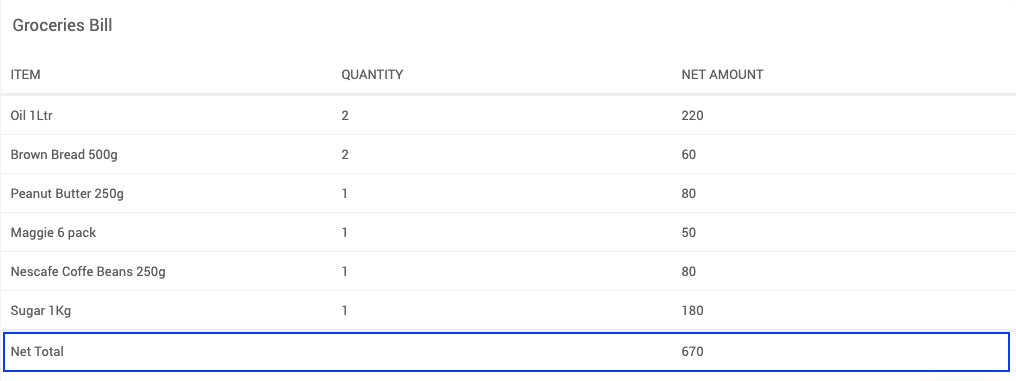### Multiple Summary Rows​

Call setSummaryRowData on the column where you want to set the summary rows data and pass an array of values.

``Page.GroceriesTable1Beforedatarender = function(widget, data, columns) {    columns.item.setSummaryRowData([        'Net Total',        'Discount'    ]);    columns.netAmount.setSummaryRowData([        '670',        '2%'    ]);};``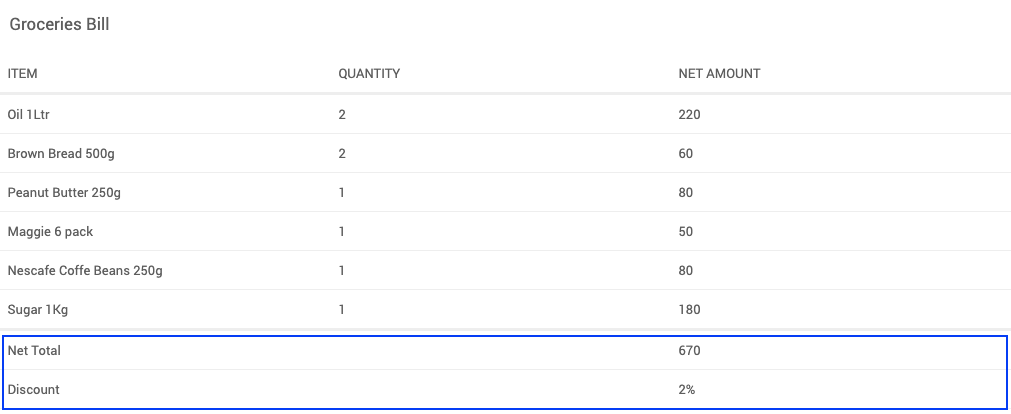### Summary Row with Aggregate Function and Variables​

Create an aggregate function instance and call the inbuilt aggregate functions on the column setSummaryRowData.

``Page.GroceriesTable1Beforedatarender = function(widget, data, columns) {    const DISCOUNT = Page.Variables.Discount.dataSet.dataValue;    const netAmountAggregate = columns.netAmount.aggregate;    columns.item.setSummaryRowData([        'Net Total',        'Discount'    ]);    columns.netAmount.setSummaryRowData([        netAmountAggregate.sum(),        DISCOUNT + '%'    ]);};``### Built In Aggregate functions​

A Column contains the below built-in aggregate functions that you can use in summary rows.

FunctionParameters
sumNA
averageNA
countNA
minimumNA
maximumNA
percentTotal value to calculate percentage

The above aggregate functions can be accessed using the `aggregate` object on the columns as shown below.

``Page.<TableName>Beforedatarender = function(widget, data, columns) {    const columnAggregate = columns.<columnName>.aggregate;    columns.<columnName>.setSummaryRowData(columnAggregate.sum());};``

## Custom Functions with aggregation logic​

### Summary Row Custom Function​

Call custom function and return data in setSummaryRowData on the column where you want to set the summary row data.

``Page.GroceriesTable1Beforedatarender = function(widget, data, columns) {    const DISCOUNT = Page.Variables.Discount.dataSet.dataValue;    const netAmountAggregate = columns.netAmount.aggregate;    columns.item.setSummaryRowData([        'Net Total',        'Discount',        'Total'    ]);    columns.netAmount.setSummaryRowData([        netAmountAggregate.sum(),        DISCOUNT + '%',        calculateTotal()    ]);    function calculateTotal() {        let total = netAmountAggregate.sum();        return total - ((total / 100) * DISCOUNT);    }};``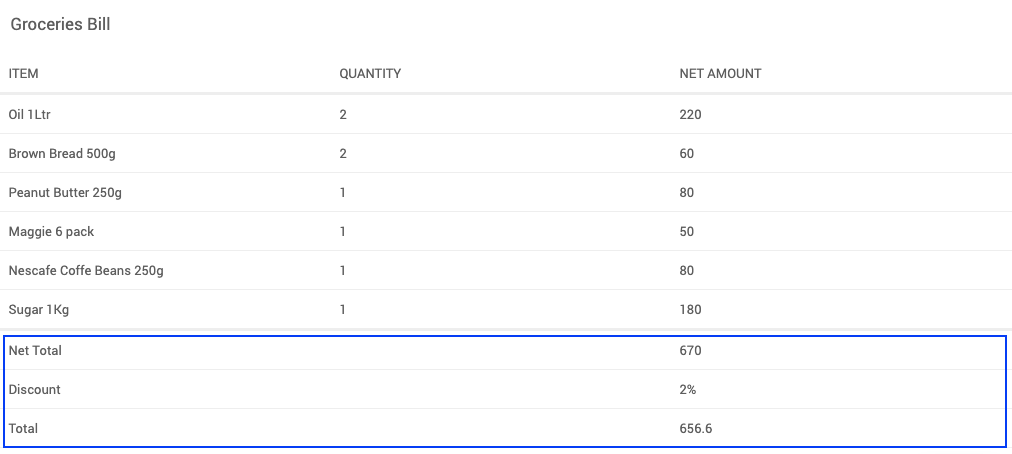## Styling the Summary row​

Return an object with keys value and class to display data and add styles associated to that class in setSummaryRowData on the column you want to set the summary row data.

``Page.GroceriesTable1Beforedatarender = function(widget, data, columns) {    const DISCOUNT = Page.Variables.Discount.dataSet.dataValue;    const netAmountAggregate = columns.netAmount.aggregate;    columns.item.setSummaryRowData([        'Net Total',        'Discount',        {            value: 'Total Budget',            class: 'bold-class'        }    ]);    columns.netAmount.setSummaryRowData([        netAmountAggregate.sum(),        DISCOUNT + '%',        {            value: calculateTotal(),            class: 'bold-class'        }    ]);    function calculateTotal() {        let total = netAmountAggregate.sum();        return total - ((total / 100) * DISCOUNT);    }};``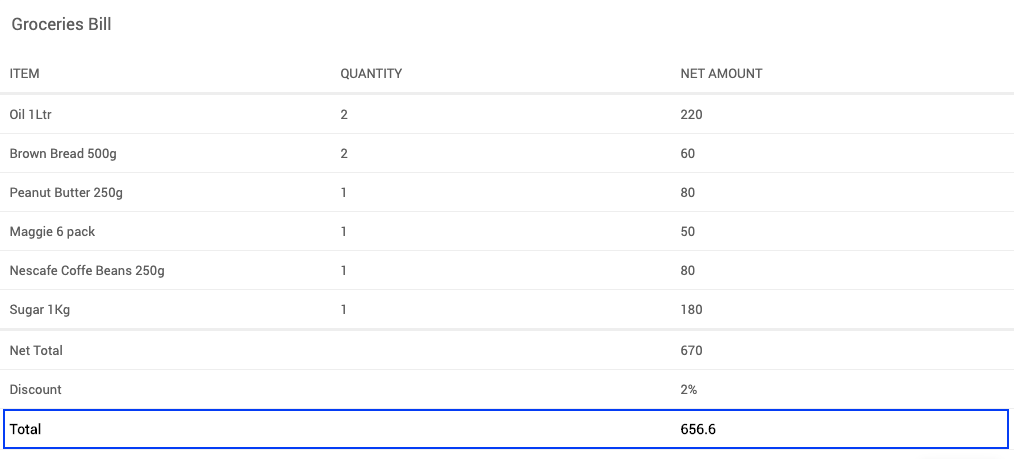You can also create a CSS class with all the required CSS properties inside the styles tab and use that class inside the `setSummaryRowData` function in the script page.

Refer below example:

``.styleSummaryRow{color: red;font-weight: bold;}``
``columns.age.setSummaryRowData({        value: 'Total Salary',        class: 'styleSummaryRow'    })};``

### Summary Row Custom Asynchronous Function​

The javascript function that calculates the summary can invoke an API to return the result of the calculation executed on the server-side. This API could be the application's business logic. For example, when calculating the applied discount, business logic may assign the discount value based on the logged-in user or amount, money spent, etc. In such a case, the javascript function will return a `Promise` after calling the API.

Here is an example of a custom javascript function that invokes an API and returns a promise to the `setSummaryRowData`.

``Page.GroceriesTable1Beforedatarender = function(widget, data, columns) {    const DISCOUNT = Page.Variables.Discount.dataSet.dataValue;    const netAmountAggregate = columns.netAmount.aggregate;    columns.item.setSummaryRowData([        'Net Total',        'Discount',        'Total'    ]);    columns.netAmount.setSummaryRowData([        netAmountAggregate.sum(),        DISCOUNT + '%',        calculateTotal()    ]);    function calculateTotal() {        return new Promise(function(resolve, reject) {            Page.Variables.Total.invoke().then((data) => {                resolve(JSON.parse(data.body).budget);            });        });    }};``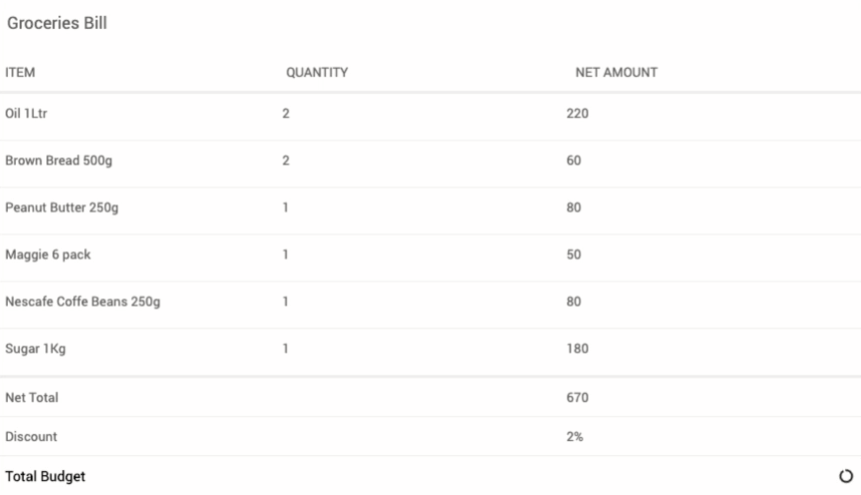## Handling Column Visibility​

note

The Summary row-column visibility may depend on the actual column's visibility. If a column is set to not show in mobile/desktop devices, the respective summary row columns may also need to be hidden. Using the authorization setting in WaveMaker, a column could be hidden for specific roles of users.

To Handle column visibility, check the column's occurrence in runtime to handle such scenarios. If a column is hidden, it will not be available under the columns interface in runtime. In such cases, consider the below code:

``Page.DepartmentTable1Beforedatarender = function(widget, data, columns) {    const budgetAggregate = columns.budget.aggregate;    columns.budget.setSummaryRowData(budgetAggregate.sum());    if (columns.deptId) {        columns.deptId.setSummaryRowData("Total Budget");    } else {        columns.name.setSummaryRowData("Total Budget");    }};``

Here, if the column `deptId` is present in the table, the label "Total Budget" is rendered under the respective column. Otherwise, it renders under the `name` column.

The scenario could suit a requirement where the column `deptId` is present in the larger screens but not in mobile screens, while column `name` is present in all the screens.

Here is how the summary row will appear in respective screens:

### Large Screen​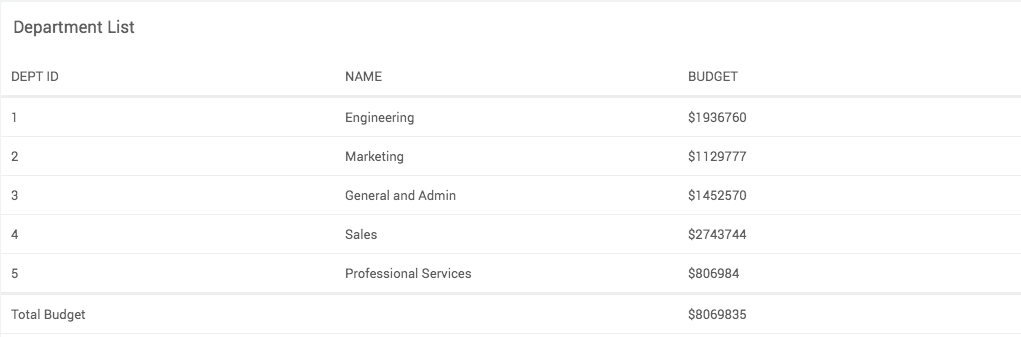### Mobile Screen​